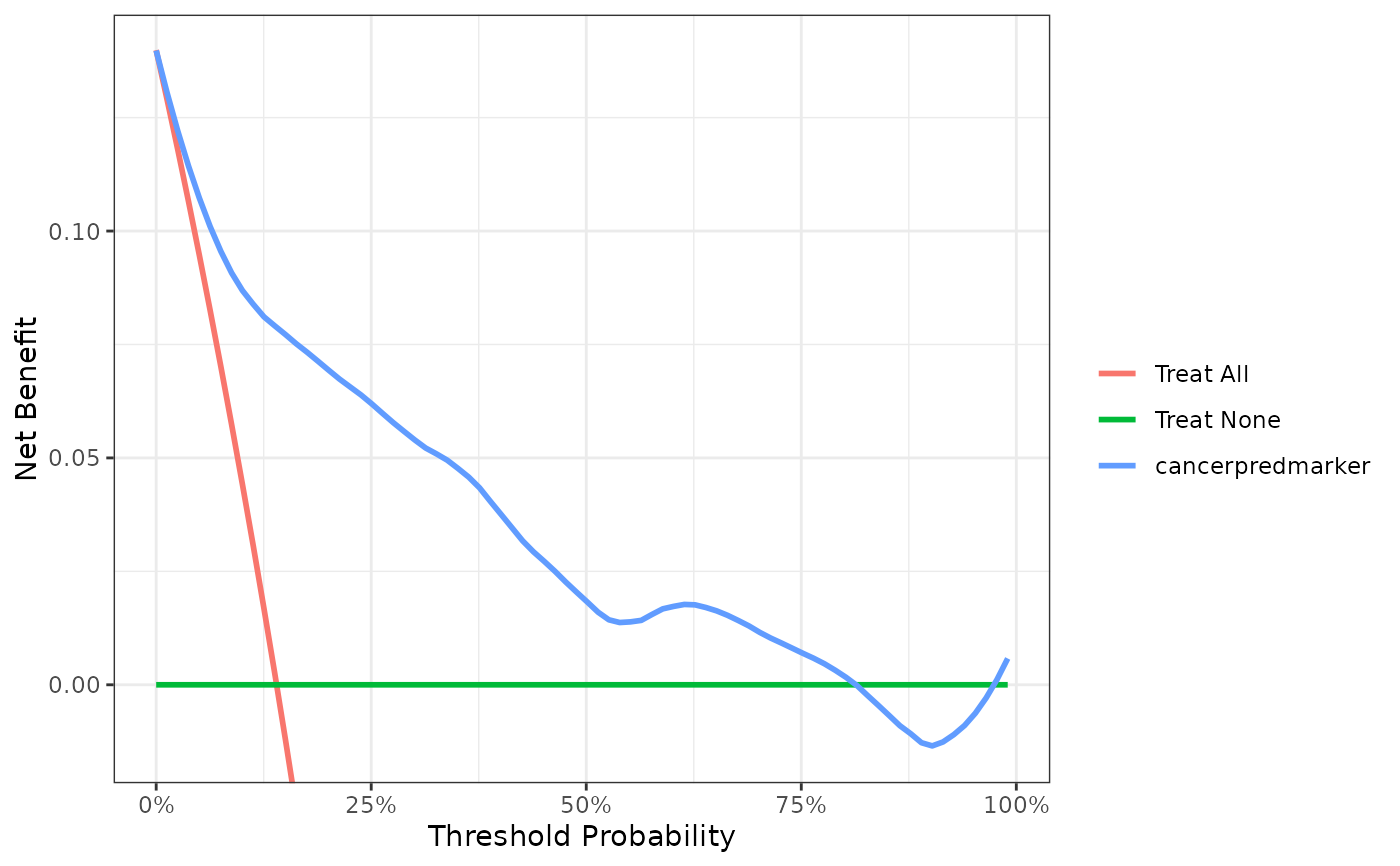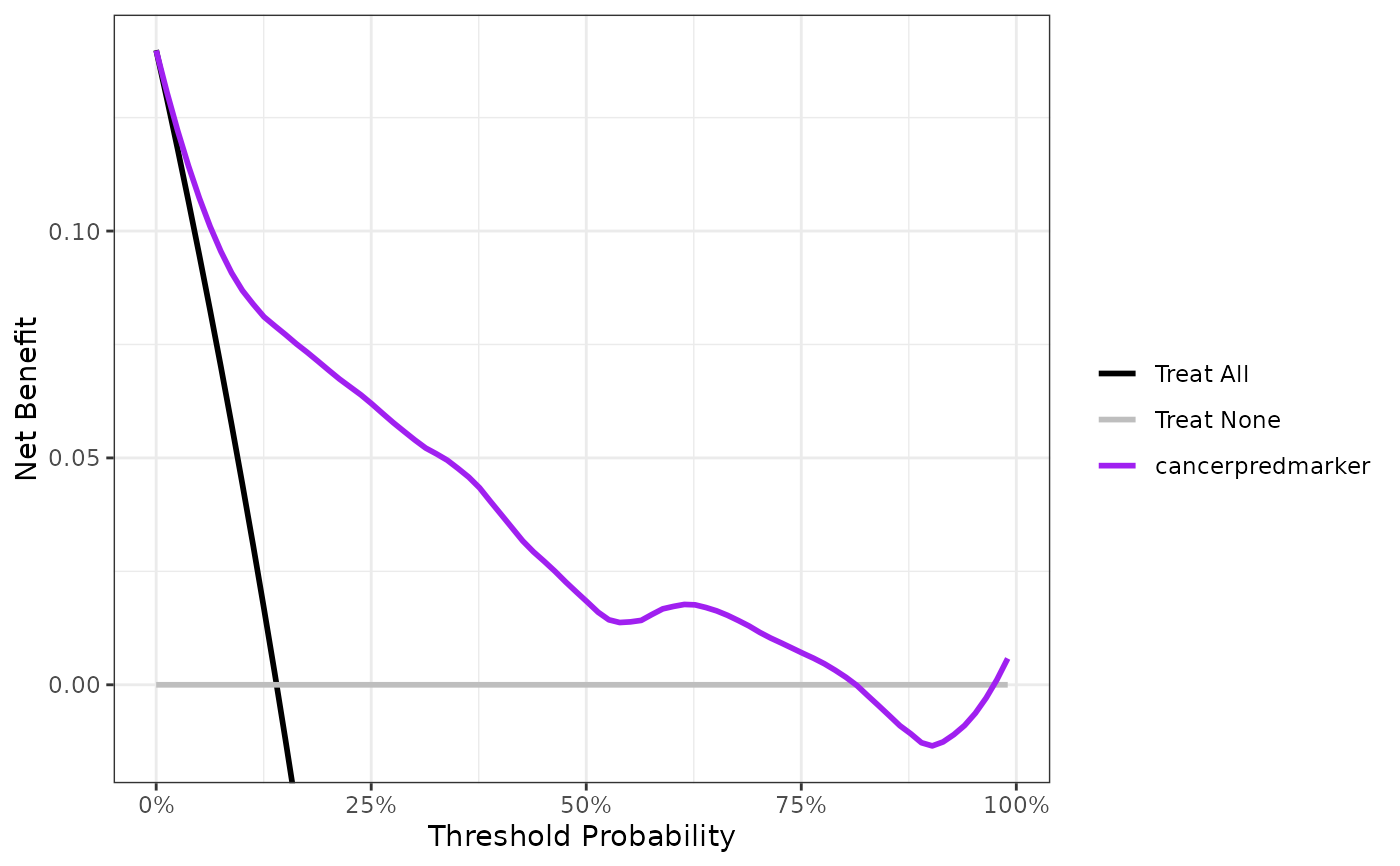Plot DCA Object with ggplot

# S3 method for dca
plot(
x,
type = NULL,
smooth = FALSE,
span = 0.2,
style = c("color", "bw"),
show_ggplot_code = FALSE,
...
)

## Arguments

x

dca object created with dca()

type

indicates type of plot to produce. Must be one of c("net_benefit", "net_intervention_avoided", "standardized_net_benefit"). The default is "net_benefit", unless the net intervention has been calculated when "net_intervention_avoided" is used, or if "standardized_net_benefit" has been calculated.

smooth

Logical indicator whether plot will be smooth with ggplot2::stat_smooth(). Default is FALSE

span

when smooth = TRUE, Controls the amount of smoothing for loess smoother. Smaller numbers produce wigglier lines, larger numbers produce smoother lines. Default is 0.2.

style

Must be one of c("color", "bw"). Default is "color", and "bw" will print a black and white figure

show_ggplot_code

Logical indicating whether to print ggplot2 code used to create figure. Default is FALSE. Set to TRUE to perform advanced figure customization

...

not used

## Value

a ggplot2 object

dca(), net_intervention_avoided(), standardized_net_benefit(), as_tibble.dca()

Daniel D Sjoberg

## Examples

p <-
dca(cancer ~ cancerpredmarker, data = df_binary) %>%
plot(smooth = TRUE, show_ggplot_code = TRUE)
#> # ggplot2 code to create DCA figure -------------------------------
#> as_tibble(x) %>%
#>   dplyr::filter(!is.na(net_benefit)) %>%
#>   ggplot(aes(x = threshold, y = net_benefit, color = label)) +
#>   stat_smooth(method = "loess", se = FALSE, formula = "y ~ x",
#>     span = 0.2) +
#>   coord_cartesian(ylim = c(-0.014, 0.14)) +
#>   scale_x_continuous(labels = scales::percent_format(accuracy = 1)) +
#>   labs(x = "Threshold Probability", y = "Net Benefit", color = "") +
#>   theme_bw()
p# change the line colors
p + ggplot2::scale_color_manual(values = c('black', 'grey', 'purple'))